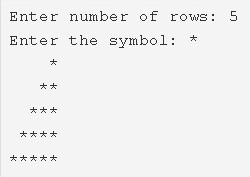# C Mirrored Right Triangle Star Pattern Program – Pattern Programs

C program to print a mirrored right triangle star pattern – In this article, we will describe the multiple ways to print a mirrored right triangle star pattern in C programming.

Suitable examples and sample programs have also been added so that you can understand the whole thing very clearly. The compiler has also been added with which you can execute it yourself.

Mirrored right triangle star pattern program in 3 Different ways:

• Using For Loop
• Using While Loop
• Using Do-While Loop

It is completely well known that a right triangle is a triangle which has a single angle equal to 90 degrees.

The sum of squares of the side-length of the adjacent sides of the right angle is equal to the square of the side opposite to the right angle.As it is evident from the image uploaded above, you have to enter the number of rows accordingly.

The number of rows entered for this example is 5.

Then, you have to select the symbol with which you want to print your Mirrored Right Triangle Pattern.

As per the title name itself, the ‘*’ pattern is chosen.

Hence, the Mirrored Right Triangle Star Pattern is printed as per the code.

Thus, the different ways to do the same in C programming are as follows:

## Using For Loop

1. Read the number of rows and entered a character, store the values into the variable n, ch.

2) The outer for loop iterates through rows from i=1 to n with the structure for(i=1;i<=n;i++).

a) The 1st inner for loop iterates through columns with the structure for(j=0;j<n-i;j++)and prints n-i spaces for each row.

b) The 2nd inner for loop iterates through columns with the structure for(j=0;j<i;j++)and prints i symbols for each row.

5) Each iteration of outer loop cursor comes to the next line.

Output:

### Using While Loop

1. The outer while loop iterates through rows until the condition i<=n becomes false.

2) The 1st inner while loop iterates through columns until the condition j<(n-i).

Prints space and j value increased by 1.

3) The 2nd inner while loop iterates through columns until the condition j<i.

Prints symbol and j value increased by 1.

4) Cursor comes to next line.

5) i value increased by 1.

Output:

#### Using Do-While Loop

1. The outer do-while loop iterates through rows.

2) The 1st inner do-while loop iterates through columns.

Prints space,j value increased by 1.

Checks the condition j<n-i. If the condition is true then the loop iterates again. Repeats until the condition becomes false.

3) The 2nd inner do-while loop iterates through columns.

Prints symbol,j value increased by 1.

Checks the condition j<i. If the condition is true then loop iterates again. Repeats until the condition becomes false.

4) Cursor comes to next line.

5) i value increased by 1.

Checks the condition i<n. If the condition is true then loop iterates again. Repeats until the condition becomes false.

Output:

x

## C Program To Print Number Of Days In A Month | Java Tutoring

C program to input the month number and print the number of days in that ...